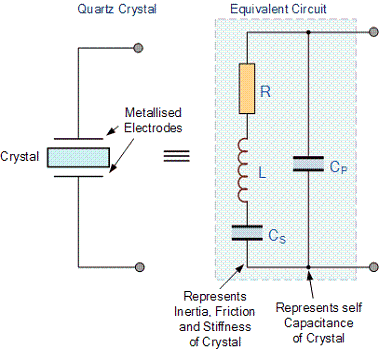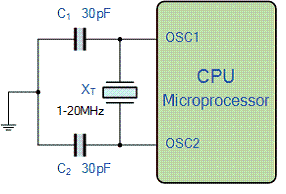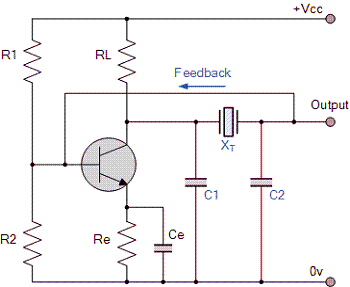0

Working of Transistor as an oscillator and amplifier

• Working of Transistor as an oscillator

Since a transistor mainly transfers the resistance, it is called as transistor.

There exists an input circuit with a small source of power and a small resistance and an output circuit with a high resistance and moderately large source of power.

In the input circuit, whenever there is a small change in current, there is an amplified variation of current in the output circuit, mainly because the resistances are transferred.

The amplified output current also oscillates with respect to the input, When the input current is made to oscillate by applying a signal to the input terminal,

How a transistor works as an amplifier.

Analogy: If we shake the pole here and there, just fix a long wooden pole on the ground. We can monitor that the top of the pole is shaking dynamically.

An amplifier can be made an oscillator by a simple way.

When the transistor is working as an amplifier, a small portion of the out power (oscillating current) is taken and it is feed to the input section. Simultaneously remove the input signal which we used for the amplification process.

For the amplifier, the input signal is got from its output and that is amplified. Also a portion of the output is again sent to the input and the process is continued. This is how the amplifier now works as an oscillator.

For the proper function of oscillator, a proper portion of the output and the phase of output is important.
0

Crystal Oscillators and Applications

• A mechanical or electronic device that works on the principles of oscillation is an oscillator. In other words, oscillator can be defined as the periodic fluctuations between two things based on changes in energy. The practical applications of oscillators include Computers, clocks, watches, radios etc.
An example for simple type of mechanical oscillator is a clock pendulum. According to the oscillation within atoms, the atomic clock keeps time. In order to generate signals in computers, wireless receivers and transmitters and audio-frequency equipments, electronic oscillators are mainly used. Particularly it is used in music synthesizers. Different types of electronic oscillators are available. All the electronic oscillators operate according to the same basic principle. An oscillator always employs a sensitive amplifier, whose output signal is fed back to the input signal in phase. Hence, the signal itself regenerates and sustains. This is called as a positive feedback. Thus the oscillator uses a positive feedback for working. This is almost same to the unwanted "howling" in public-address systems.

A quartz crystal determines the frequency at which an oscillator works. When a direct current is applied, these crystals vibrate at a frequency that depends on its thickness value and on the manner in which it is cut from the original mineral rock. To determine the frequency, some oscillators employ combinations of inductors, resistors, and capacitors. But, the use of quartz crystals gives the best stability (constancy of frequency) in oscillators.

In a computer the clock serves as a sort of pacemaker for the microprocessor. The clock is nothing but a specialized oscillator.  The clock frequency (also called as clock speed) is usually specified in megahertz (MHz) frequency. The clock frequency is an important factor in determining the rate at which a computer can perform the execution of instructions.Figure 1
Figure 1 shows the equivalent electronic circuit diagram of a crystal. The equivalent diagram of a crystal consists of a resistor, an inductor and two capacitors. The two capacitors are named as Cs and Cp.Figure 2
Figure 2 shows the circuit diagram of a crystal, which is used as a clock for the microprocessor used in a CPU..Figure 3
Figure 3 shows the equivalent circuit diagram of an oscillator constructed by a Field Emitter Transistor (FET).Figure 4
Figure 4 shows the equivalent circuit diagram of an oscillator constructed by a Bipolar Junction Transistor (BJT). In the circuit, Xt denotes the crystal.